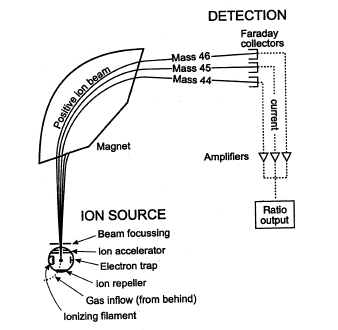## Mass Spectrometry to Measure Mass

#### Learning Objective

• Define the primary application of a mass spectrometer

#### Key Points

• Mass spectrometers work on samples in a gaseous state.
• The gaseous samples are ionized by an ion source, which adds or removes charged particles (electrons or ions). Examples of ion sources include inductively coupled plasma and electron impact.
• Mass analyzers separate ionized samples according to their mass-to-charge ratio. Time-of-flight and quadrupole are examples of mass analyzers.
• A particle’s mass can be calculated very accurately based on parameters such as how long it takes to travel a certain distance or its angle of travel.
• Mass spectrometers are so accurate that they can determine the types of elements in a compounds or measure the differences between the mass of different isotopes of the same atom.

#### Terms

• ionizationAny process that leads to the dissociation of a neutral atom or molecule into charged particles (ions).
• plasmaA state of matter consisting of partially ionized gas, usually at high temperatures.
• mass-to-charge ratioThe best way to separate ions in a mass spectrometer. This number is calculated by dividing the ions weight by its charge.

Mass spectrometry (MS) is a powerful technique that can identify a wide variety of chemical compounds. It is used to determine a particle’s mass, the elemental composition of a sample, and the chemical structures of larger molecules.

Mass spectrometers separate compounds based on a property known as the mass-to-charge ratio: the mass of the atom divided by its charge. First, the sample is ionized. Ionization is the process of converting an atom or molecule into an ion by adding or removing charged particles such as electrons or ions. Once the sample is ionized, it is passed through some form of electric or magnetic field. A particle’s mass can be calculated based on parameters such as how long it takes to travel a certain distance or its angle of travel.Schematic of Mass SpectrometerA sample is loaded onto the mass spectrometer, where it undergoes vaporization and ionization. The components of the sample are ionized by one of a variety of methods, such as the ionizing filament. The ions are separated in an analyze by magnetic fields. They are separated according to their mass-to-charge ratios. The ions are detected, usually by a quantitative method such as a Faraday collector. The ion signal is processed into a mass spectrum.

## The Make-Up of Mass Spectrometry (MS) Instruments

MS instruments consist of two main components:

• An ion source, which can convert sample molecules into ions
• A mass analyzer, which sorts the ions by mass by applying electromagnetic fields

There are a wide variety of techniques for ionizing and detecting compounds.

## Ionizing Compounds

The ion source is the part of the mass spectrometer that ionizes the compound. Depending on the information desired from mass spectrometry analysis, different ionization techniques may be used. For example, the most common ion source for analyzing elements is inductively coupled plasma (ICP). In ICP, a 10,000-degree C “flame” of plasma gas is used to atomize sample molecules and strip the outer electrons from those atoms.

The plasma is usually generated from argon gas. Plasma gas is electrically neutral overall, but a substantial number of its atoms are ionized by the high temperature.

Electron impact (EI) is another method for generating ions. In EI, the sample is heated until it becomes a gas. It is then passed through a beam of electrons. This high-energy beam strips electrons from the sample molecules, leaving behind a positively charged radical species.

## Mass Analyzers

Mass analyzers separate the ions according to their mass-to-charge ratios. There are many types of mass analyzers. Each has its strengths and weaknesses, including:

• how accurately they can measure similar mass-to-charge ratios
• the range of masses and sample concentrations they can measure.

For example, a time-of-flight (TOF) analyzer uses an electric field to accelerate the ions through the same potential and then measures the time they take to reach the detector. Since the particles all have the same charge, their velocities depend only on their masses, and lighter ions will reach the detector first.